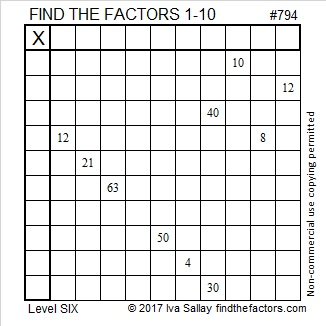# 794 and Level 6

794 is the hypotenuse of a Pythagorean triple, 456-650-794, so 456² + 650² = 794².

794 is also palindrome 282 in BASE 18. Note that 2(18²) + 8(18) + 2(1) = 794.

Stetson.edu informs us that 1^6 + 2^6 + 3^6 = 1 + 64 + 729 = 794.Print the puzzles or type the solution on this excel file: 10-factors-788-794

————————————–

• 794 is a composite number.
• Prime factorization: 794 = 2 x 397
• The exponents in the prime factorization are 1 and 1. Adding one to each and multiplying we get (1 + 1)(1 + 1) = 2 x 2 = 4. Therefore 794 has exactly 4 factors.
• Factors of 794: 1, 2, 397, 794
• Factor pairs: 794 = 1 x 794 or 2 x 397
• 794 has no square factors that allow its square root to be simplified. √794 ≈ 1780056.————————————–